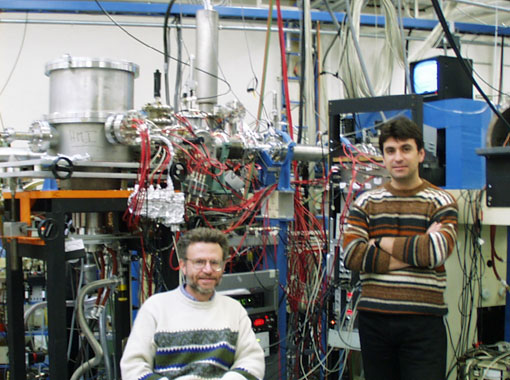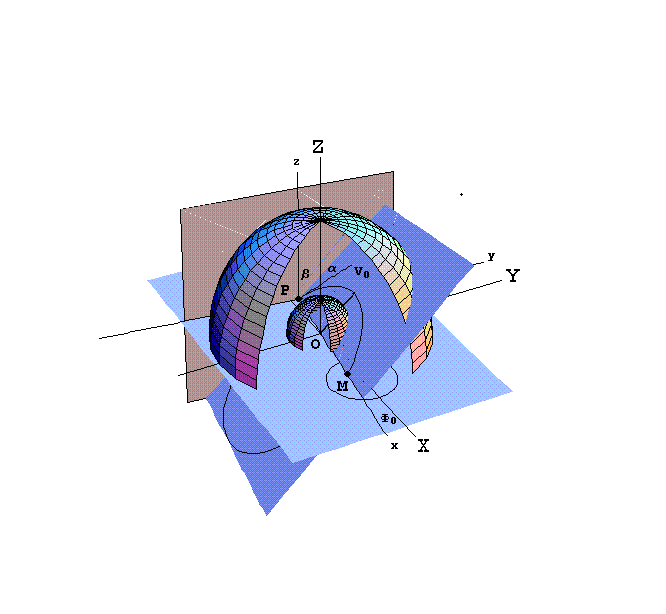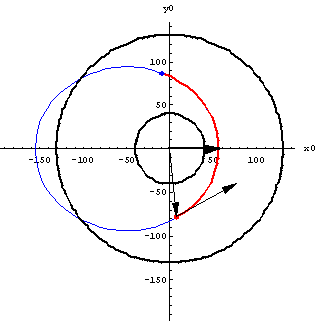## Welcome to the homepage of  Theo J.M. ZourosZERO-DEGREE AUGER SPECTROSCOPY
of HIGHLY CHARGED PROJECTILE IONS

Above: a view of the University of Crete experimental setup
at the 7 MV tandem accelerator facility at Kansas State University

On the left can be seen the experimental setup including collision chamber and beamline, housing the new zero-degree hemispherical spectrograph. In the front former University of Crete graduate student (PhD 2001) Manolis Benis (on the right) and Prof. Theo Zouros.

Electron orbits in a Hemispherical Deflector Analyser3D schematic of an electron orbit in a hemispherical spectrometer designed with Mathematica. The definition of the trajectory angles α and β are clearly shown as well as the plane of the orbit. In this picture α=-30o and β=-50o Particle enters spectrometer at point P and exits at point M after going through a deflection of 180 degrees. The XYZ-frame is the fixed spectrometer frame, while the xyz-frame is the relative trajectory frame. Both Z- and z- axes are always parallel. The short dark line starting at O is the eccentricity vector ε which is conserved.Trajectory of above electron (red line) is part of an ellipse. The bold arrow along the x0 axis is the eccentricity vector ε, which is conserved. The two black circles are the inner and outer radii of the analyzer. The arrow pointing to the red dot is the position vector, while the arrow pointing away from the red dot and tangent to the ellipse is the velocity vector.